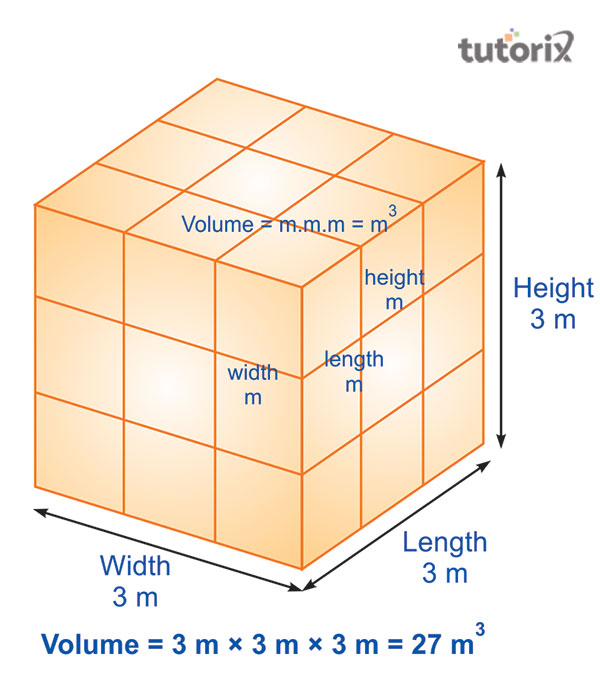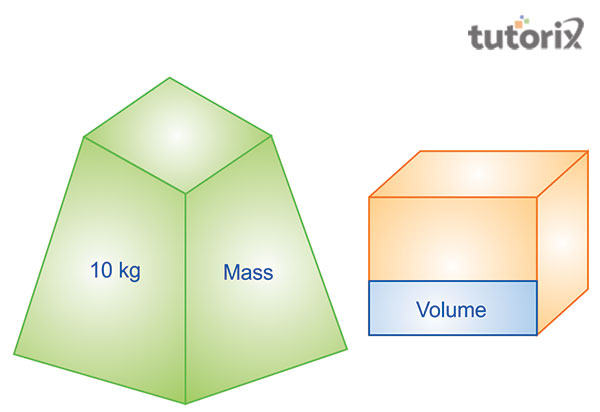# Difference Between Mass and Volume

## Introduction

Mass and Volume are two important aspects of physics. The former mainly defines the amount of matter within an object’s body. The latter one is nothing but the space taken by the object. The main difference between these two aspects is that volume is the geometric value and mass is a physical value of an object. The differences between these two matters say that they have different values and both of the matters have a different state in which they react.

## What is Mass?

Mass mainly defines the quantity of matter within a solid particle. It is considered the measurement of inertia of an object. It can be measured through milligrams and grams and its SI unit is Kilogram. The matter of mass defines the factor that it is a physical value (Werner et al. 2020).

The mass has a direct relationship with the gravitational force. The matter of mass also says every substance is made of matter and mass is the quantity of matter within an object. Here it is seen that if the shape of an object is changed, then it does not affect the mass. On the other hand, if a part is removed from the object then it gets affected. It is seen that mass is a dimensionless scalar quantity and it changes as per the volume and density of an object. This is the concept of mass and it is a matter’s quantity.

## What is Volume?

Volume is nothing but the space taken by a substance. Volume mainly refers to a threedimensional quantity. Its main use of it is to measure the capacity of a solid shape (Nuclear-power, 2022). Simply, it defines the amount of three-dimensional space occupied by a closed figure as measured by the volume of the figure. The measurement of volume is done through cubic units like cubic millimetres, cubic centimetres, and cubic meters. The SI unit of a volume is cubic meter.Figure 1: Volume

The volume of the sphere is measured by using the formula V = 4/3πr3. Here r can be considered as the radius of the sphere. A square having 3 meters of the side will have a volume of 27 cubic meters.

## The main differences between Mass and VolumeFigure 2: Difference between Mass and Volume

Sl.No Mass Volume
1.

Mass means the physical quantity of an object. It described the amount of matter present in the object’s body.

Volume here refers to the quantity’s evaluation based on the space of the area. Volume needs a 3-dimensional area for the presentation of the object (Askanydifference, 2022).

2.

Mass mainly defines the measurement of weight of an object.

Volume, on the other hand, refers to the measurement of a finite amount of area of the object.

3.

Mass is the regular and constant property of an object. It is seen that a body possesses some mass always (McMaster, 2019).

A substance’s volume changes with the change of an object because it depends on the substance’s state. The state of an object can be liquid, solid, or gaseous.

4.

It is seen that the mass s the physical value of an object

Volume, on the other hand, is the geometric value of an object.

5.

The mass of a liquid can get through the weight of a container with the liquid.

The volume can get through the measurement of the length, depth, and width of the container.

Table 1: Difference between Mass and Volume

## Other differences between the mass and volume

Mass of an object and the volume of an object are different from each other. It is seen that after having the above-mentioned differences, these factors also have some other differences. The mass of an object does not depend on the state of the object but volume differs as per the state of an object. Mass with the equation helps to convert energy and it helps in the chemical reaction. Volume does not have the capacity to do that. Mass rises with the rise of velocity while it is the opposite in the case of volume.

## Conclusion

The aspects of mass and volume have differences and the change in the number of objects affects both the matters. It is seen that the mass of an object increased when the object lies in motion. Mass here is the physical quantity of an object whereas volume is the evaluation of quantity of an object. In the case of volume, a 3-dimensional area is required for the object. The capacity of the volume changes in the change of the state of the object. Therefore, it is said that both the aspects of volume and mass have great differences in the case of objects.

## FAQs

Q1. What is the major difference between volume and mass?

Ans. Volume is the measurement of the area of an object whereas mass is the matter that an object contains within itself. Here, both of the matters have different measurement units.

Q2. What is the SI unit of volume and mass?

Ans. The SI unit of volume is cubic meters and the SI unit of mass is Kilogram. Cubic meters is represented as m3 and Kilogram is written as kg.

Q3. What does density refer?

Ans. The density of a body is nothing but the amount of mass per unit volume. Density has a common symbol and it is applied to denote density. The symbol is p and density is defined as the degree of compactness of a substance.

Q4. What are the examples of volume and mass?

Ans. Volume is considered the geometric value whereas mass is the physical value of an object. Here, the volume of a pencil and pen seems similar but their weights vary and it is a common example of these matters.

Updated on: 13-Oct-2022

1K+ Views